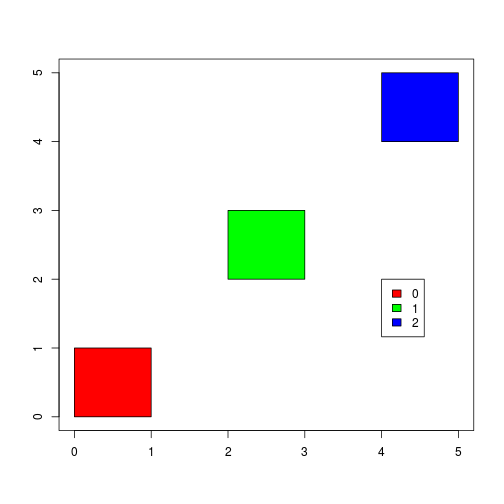Want to share your content on R-bloggers? click here if you have a blog, or here if you don't.

### Introduction

The purpose of this post is to show how to use Boost::Geometry library which was introduced recently in Rcpp. Especially, we focus on R-tree data structure for searching objects in space because only one spatial index is implemented – R-tree Currently in this library.

Boost.Geometry which is part of the Boost C++ Libraries gives us algorithms for solving geometry problems. In this library, the Boost.Geometry.Index which is one of components is intended to gather data structures called spatial indexes which are often used to searching for objects in space quickly. Generally speaking, spatial indexes stores geometric objects’ representations and allows searching for objects occupying some space or close to some point in space.

### R-tree

R-tree is a tree data structure used for spatial searching, i.e., for indexing multi-dimensional information such as geographical coordinates, rectangles or polygons. R-tree was proposed by Antonin Guttman in 1984 as an expansion of B-tree for multi-dimensional data and plays significant role in both theoretical and applied contexts. It is only one spatial index implemented in Boost::Geometry.

As a real application of this, It is often used to store spatial objects such as restaurant locations or the polygons which typical maps are made of: streets, buildings, outlines of lakes, coastlines, etc in order to perform a spatial query like “Find all stations within 1 km of my current location”, “Let me know all road segments in 2 km of my location” or “find the nearest gas station” which we often ask google seach by your voice recenlty. In this way, the R-tree can be used (nearest neighbor) search for some places.

You can find more explanations about R-tree in Wikipedia.

### Write a wrapper class of rtree in Boost::Geometry using Rcpp

Now, we write a simple C++ wrapper class of rtree class in Boost::Geometry::Index that we can use in R.

The most important feature to mention here is the use of Rcpp module to expose your own class to R. Although almost all classes in Boost library have a lot of functions, , you do not use all in many cases. In that case, you should write your wrapper class for making your code simple.

### Rcpp code

```// [[Rcpp::depends(BH)]]

// Enable C++11 via this plugin to suppress 'long long' errors
// [[Rcpp::plugins("cpp11")]]

#include <vector>
#include <Rcpp.h>
#include <boost/geometry.hpp>
#include <boost/geometry/geometries/point.hpp>
#include <boost/geometry/geometries/box.hpp>
#include <boost/geometry/index/rtree.hpp>
using namespace Rcpp;

// Mnemonics
namespace bg = boost::geometry;
namespace bgi = boost::geometry::index;
typedef bg::model::point<float, 2, bg::cs::cartesian> point_t;
typedef bg::model::box<point_t> box;
typedef std::pair<box, unsigned int> value_t;

class RTreeCpp {
public:
// Constructor.
// You have to give spatial data as a data frame.
RTreeCpp(DataFrame df) {
int size = df.nrows();
NumericVector id   = df;
NumericVector bl_x = df;
NumericVector bl_y = df;
NumericVector ur_x = df;
NumericVector ur_y = df;
for(int i = 0; i < size; ++i) {
// create a box
box b(point_t(bl_x[i], bl_y[i]), point_t(ur_x[i], ur_y[i]));
// insert new value
rtree_.insert(std::make_pair(b, static_cast<unsigned int>(id[i])));
}
}
// This method(query) is k-nearest neighbor search.
// It returns some number of values nearest to some point(point argument) in space.

std::vector<int> knn(NumericVector point, unsigned int n) {
std::vector<value_t> result_n;
rtree_.query(bgi::nearest(point_t(point, point), n), std::back_inserter(result_n));
std::vector<int> indexes;
std::vector<value_t>::iterator itr;
for ( itr = result_n.begin(); itr != result_n.end(); ++itr ) {
value_t value = *itr;
indexes.push_back( value.second );
}
return indexes;
}
private:
// R-tree can be created using various algorithm and parameters
// You can change the algorithm by template parameter.
// In this example we use quadratic algorithm.
// Maximum number of elements in nodes in R-tree is set to 16.
};

// [[Rcpp::export]]
std::vector<int> showKNN(Rcpp::DataFrame df, NumericVector point, unsigned int n) {
RTreeCpp tree(df);            // recreate tree each time
return tree.knn(point, n);
}```

### R code using RTreeCpp

First, we create a sample data set of spatial data.

```# Sample spatial data(boxes)
points <- data.frame(
id=0:2,
bl_x=c(0, 2, 4),
bl_y=c(0, 2, 4),
ur_x=c(1, 3, 5),
ur_y=c(1, 3, 5))
/*
* To visually the data, we use the following code:
*/
size <- nrow(points)
#colors for rectangle area
colors <- rainbow(size)
#Plot these points
plot(c(0, 5), c(0, 5), type= "n", xlab="", ylab="")
for(i in 1:size){
rect(points[i, "bl_x"], points[i, "bl_y"], points[i, "ur_x"], points[i, "ur_y"], col=colors[i])
}
legend(4, 2, legend=points\$id, fill=colors)```One can use the RTreeCpp class as follows:

```# new RTreeCpp object
# Search nearest neighbor points(return value : id of points data)
showKNN(points, c(0, 0), 1)

 0

showKNN(points, c(0, 0), 2)

 1 0

showKNN(points, c(0, 0), 3)

 2 1 0
```

Note the re-creation of the `RTreeCpp` object is of course inefficient, but the Rcpp Gallery imposes some constraints on how we present code. For actual application a stateful and persistent object would be created. This could be done via Rcpp Modules as well a number of different ways. Here, however, we need to recreate the object for each call as `knitr` (which is used behind the scenes) cannot persist objects between code chunks. This is simply a technical limitation of the Rcpp Gallery—but not of Rcpp itself.